• Call Now

1800-102-2727•

# Lami's Theorem- Statement, Derivation, Limitation, Practice Problems, FAQs

Suppose you are hanged on a horizontal rod with both of your hands at some angle. Are you interested in finding the forces acting on your hand?

## What is Lami's Theorem?

Lamis theorem gives the relation between magnitudes of three coplanar, concurrent and nonlinear forces and angles between any two. It is helpful in analysis of various mechanical and structural systems in equilibrium.

## Lami's Theorem Statement

According to lami's theorem “When 3 forces are acting at a point in equilibrium, then each force will be proportional to the sine of angle between the other two forces ”

Let's three forces A, B and C acting on a body or particle in equilibrium and α, β and γ are the angles between them as shown in the figure. Then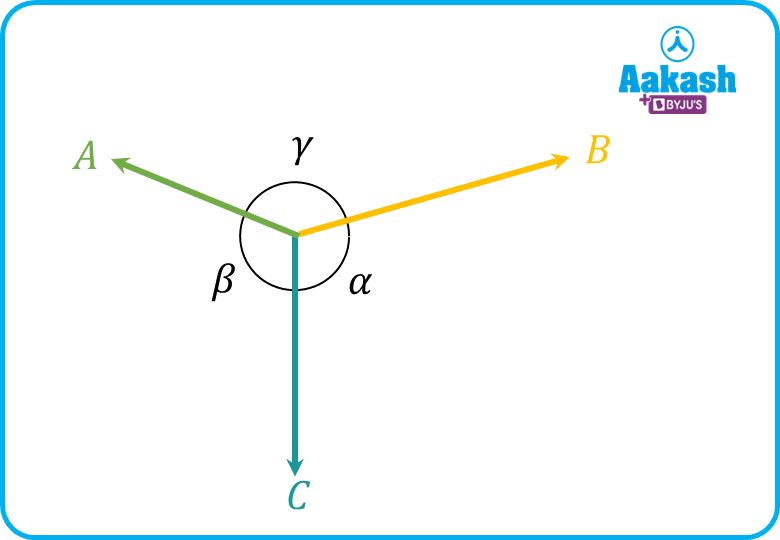Mathematically, it can express as## Lami’s Theorem Derivation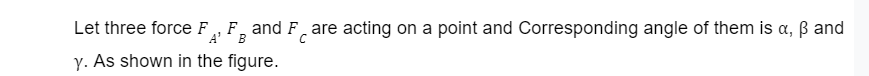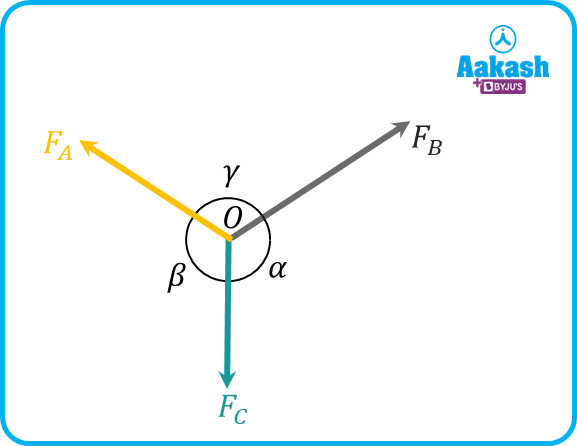As Forces are vectors they can move to form a triangle. After moving the forces and writing the angle in a complementary form,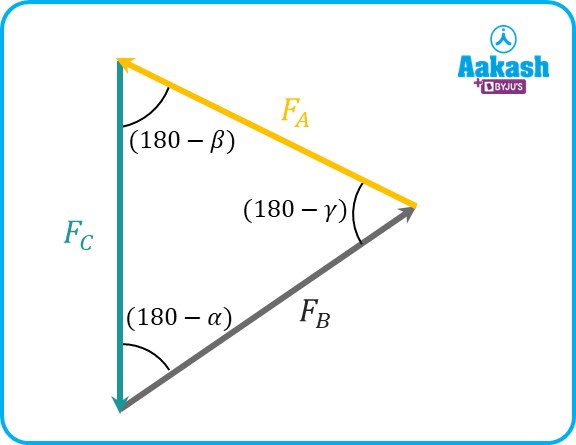Now using the Sine Rule we get,After simplifying, we haveIn general, we can writeHence Proved.

## Limitations of Lami's Theorem

• Can be applied with three forces.
• The three forces should be coplanar, which means they must lie in the same plane.
• Forces should be concurrent, which means their line of action must meet at a single point.
• Force should be nonlinear, which means angle between line of action of any of the two forces should not be 0॰ or 180॰
• Three forces should be in equilibrium.

## Practice Problems of Lami's Theorem

Question.1 A school bell of mass 30 kg is hanging from the ceiling with the help of string at point A and B as shown in the diagram. Find the tension in strings.(Take g=10 m/s2 )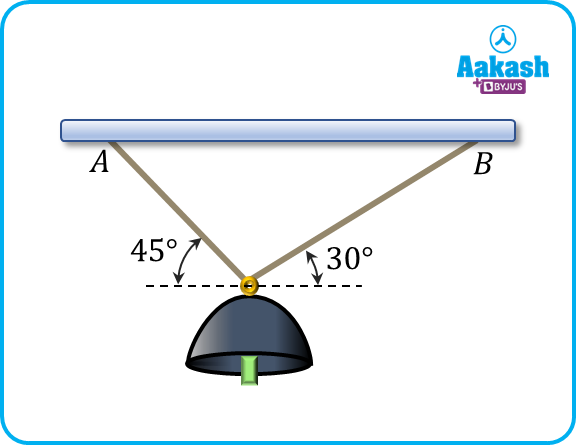Answer: Given : Mass m=30 kg

Force due to gravity on the bell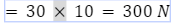Now, drawing the free body diagram of the given situation taking bell at point C,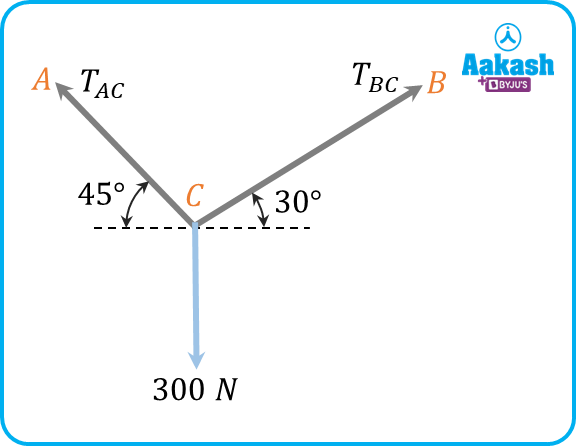Using lami's theoremPutting the values from diagramTaking first and third term,During the second and third term,

Hence, the tensions in Strings are 268.97 N and 219.61 N.

Question.2 Two persons A and B are drawing a bucket of water from a well using two ropes as shown in figure. In a given situation if person A is applying 30 N force. Find the Weight of the bucket.?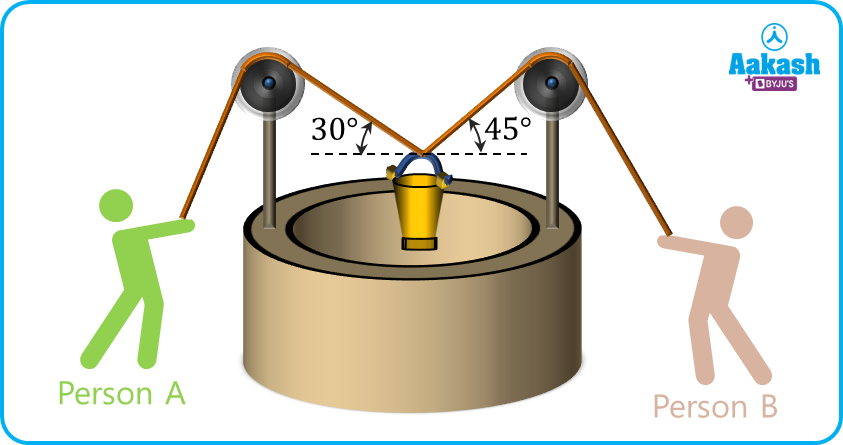Answer: Given :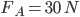Let the weight of water bucket =W

Now, draw the free body diagram of the given situation taking a bucket at point O,

Using lami's theorem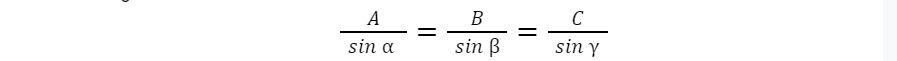Putting the values from diagramTaking first and third term,Hence, the Weight of the bucket is 33.46 N.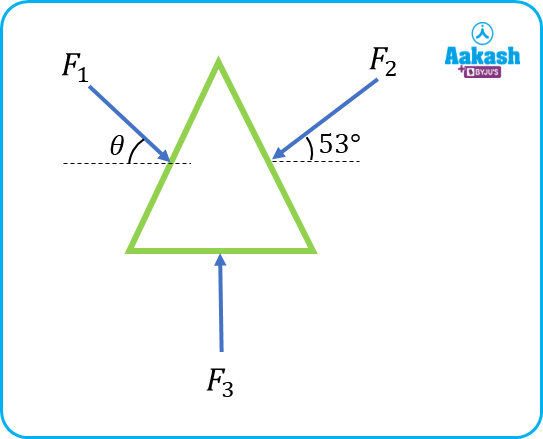Answer: Given :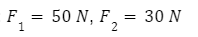Draw the free body diagram of the given situation,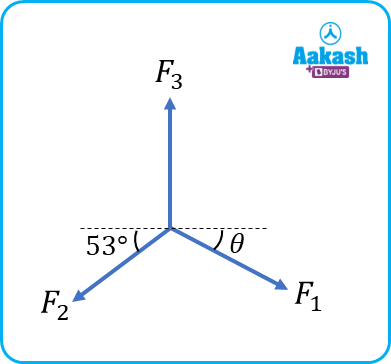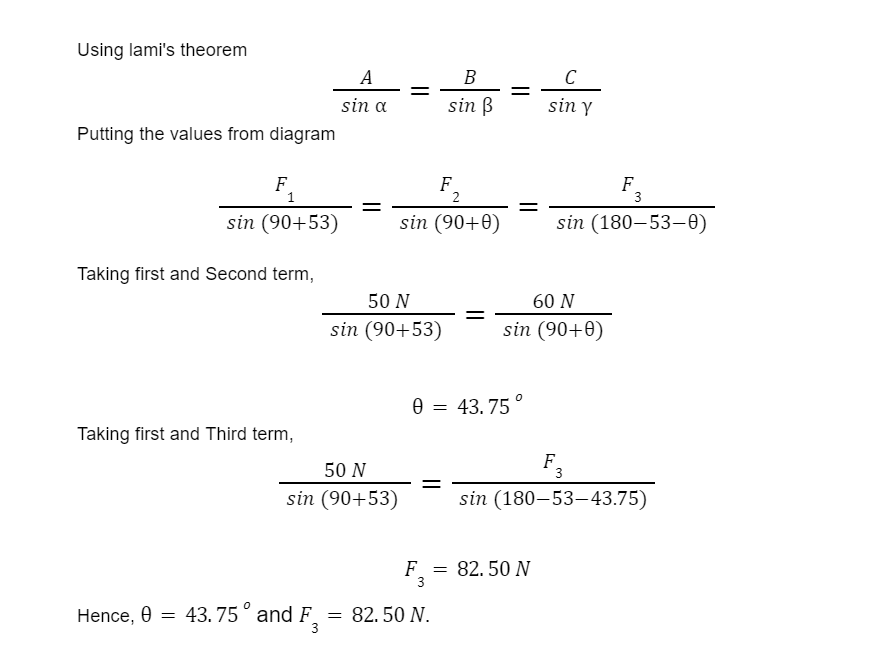Question.4 A disk of weight W=4450 N rests on a smooth inclined plane and is kept stationary with the help of a string as shown in the figure. Find tension in the rod.?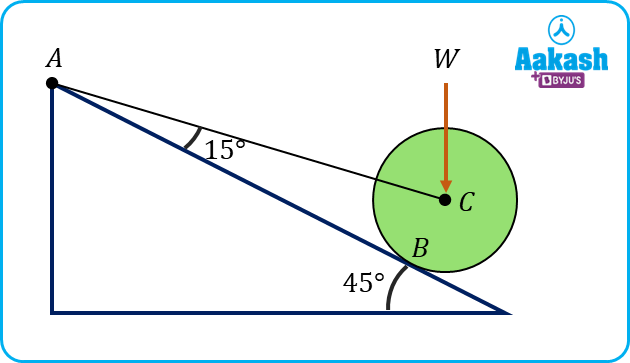Answer: Let tension in string =T

and reaction at point B =RB

Drawing the FBD of given situation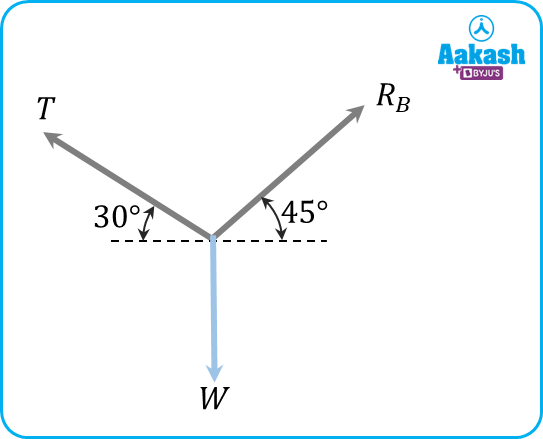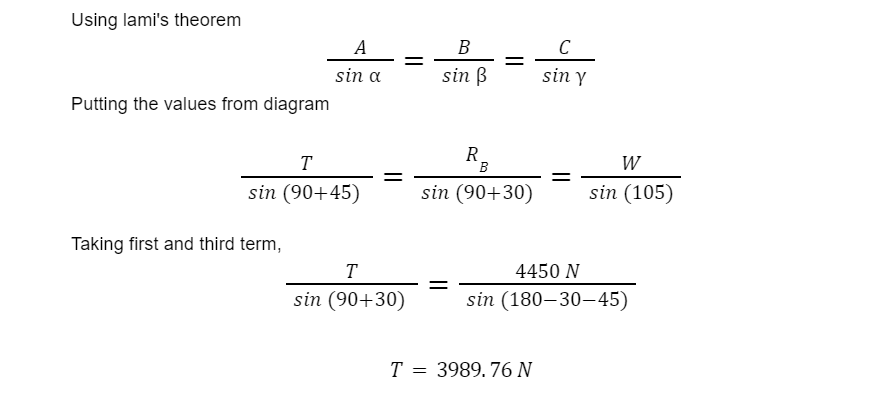Hence, the tension in the string is 3989.76 N.

## FAQs of Lami's Theorem

Question.1 What is Lami's theorem?

Answer: It is the relation between 3 coplanar, concurrent and nonlinear forces and their angles.

Question.2 What is the condition to apply Lami's theorem?

Answer: Forces should be Coplanar, concurrent and nonlinear.

Question.3 How many forces are required for the Lami'ss theorem?

Answer: three forces required for lami's theorem.

Question.4 Lami's theorem is used for?

Answer: It is used for solving various mechanical and structural problems.

## NCERT Class 11 Physics Chapters

 Physical World Units and Measurements Motion in a Straight Line Motion in a Plane Laws of Motion Work Energy and Power Particles and Rotational Motion Gravitation Mechanical Properties of Solids Mechanical Properties in Liquids Thermal Properties of Matter Thermodynamics Kinetic Theory Oscillations Waves
Talk to our expert
Resend OTP Timer =
By submitting up, I agree to receive all the Whatsapp communication on my registered number and Aakash terms and conditions and privacy policy